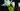# 在 JavaScript 中创建并填充任意长度的数组

``const arr = [0, 0, 0];``

## 没有空白元素的数组性能更好

``````Object.keys(['a', , 'c']);
// -> [ '0', '2' ]``````

• 字典存储，这样查找将花费更多时间，并且存储开销也更大。
• 连续的数据结构存储，带有空白的元素值。然后，检查值是否为空，将花费额外的时间。

## 创建数组

### 数组构造函数

``````const LEN = 3;
const arr = new Array(LEN);
console.log(arr.length === LEN); // true
// 数组中只有一些空白
console.log(Object.keys(arr)); // []``````

• 这些空白会使此 Array 稍慢一些，即使以后再用值完全填充它也是如此。
• 空白很少是元素的良好初始“值”。例如，零更加常见。

### 数组构造函数加 .fill() 方法

`.fill()` 方法使用指定值填充现有数组来改变它。帮助在通过 `new Array()` 创建数组后初始化数组：

``````const LEN = 3;
const arr = new Array(LEN).fill(0);
console.log(arr); // -> [0, 0, 0]``````

``````const LEN = 3;
const obj = {};

const arr = new Array(LEN).fill(obj);
console.log(arr);
// -> [{}, {}, {}]

obj.prop = true;
console.log(arr);
// -> [ {prop:true}, {prop:true}, {prop:true} ]``````

### .push() 方法

``````const LEN = 3;
const arr = [];
for (let i = 0; i < LEN; i++) {
arr.push(0);
}
console.log(arr);
// -> [0, 0, 0]``````

### 使用 undefined 填充数组

`Array.from()` 能转换迭代器和类数组值为数组。它将空白作为 `undefined` 元素。我们可以使用 `Array.from()` 转换每一个空白为 `undefined`

``````Array.from({ length: 3 });
// -> [ undefined, undefined, undefined ]``````

``````[...new Array(3)];
// -> [ undefined, undefined, undefined ]``````

### Array.from() 映射

#### 使用值填充数组

• 使用小整数创建数组
``````Array.from({ length: 3 }, () => 0);
// -> [0, 0, 0]``````
• 使用独立(非共享)对象创建对象
``````Array.from({ length: 3 }, () => ({}));
// -> [ {}, {}, {} ]``````

#### 创建整数数组

• 创建增长整数数组
``````Array.from({ length: 3 }, (x, i) => i);
// -> [(0, 1, 2)];``````
• 创建任意范围的整数
``````const START = 2,
END = 5;
Array.from({ length: END - START }, (x, i) => i + START);
// -> [ 2, 3, 4 ]``````

``````[...new Array(3).keys()];
// -> [0, 2, 3]``````

## 创建数组的备忘录

• `new Array(3)`
[ , , ,]
• `Array.from({ length: 2 })`
`[undefined, undefined]`
• `[...new Array(2)]`
`[undefined, undefined]`

• `const a = []; for (let i = 0; i < 3; i++) a.push(0);`
`[0, 0, 0]`
• `new Array(3).fill(0)`
`[0, 0, 0]`
• `Array.from({ length: 3 }, () => ({}))`
`[{}, {}, {}]` (unique objects)

• `Array.from({ length: 3 }, (x, i) => i)`
`[0, 1, 2]`
• `const START = 2, END = 5; Array.from({ length: END-START }, (x, i) => i + START)`
`[2, 3, 4]`
• `[...new Array(3).keys()]`
`[0, 1, 2]`

### 推荐的方式

• 是否需要创建不需要（或晚一点）填充的数组？
``new Array(LEN);``
• 是否需要创建使用基础类型数据初始化的数组？
``new Array(LEN).fill(0);``
• 是否需要创建使用对象初始化的数组？
``Array.from({ length: LEN }, () => ({}));``
• 是否需要创建整数数组？
``Array.from({ length: END - START }, (x, i) => i + START);``

#### 提示：数组性能通常无关紧要

• 在大多数情况下，我不用必担心性能。甚至有空白元素的数组也非常快。担心代码易于理解更有意义。
• 此外，引擎如何优化以及在何处进行优化。因此，最快的是今天而不是明天。